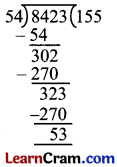# DAV Class 4 Maths Chapter 4 Worksheet 3 Solutions

The DAV Class 4 Maths Book Solutions and DAV Class 4 Maths Chapter 4 Worksheet 3 Solutions of Division offer comprehensive answers to textbook questions.

## DAV Class 4 Maths Ch 4 WS 3 Solutions

Question 1.
Divide:
(a) 7,982 ÷ 11Q = 725
R = 7

(b) 6,287 ÷ 12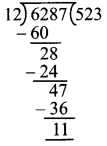Q = 523
R = 11

(c) 6,258 ÷ 25Q = 250
R = 8(d) 9,826 ÷ 37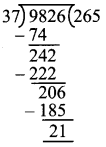Q = 265
R = 21

(e) 1,889 ÷ 62Q = 30
R = 29

(f) 5,985 ÷ 75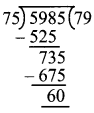Q = 79
R = 60

Question 2.
(a) 1,826 by 12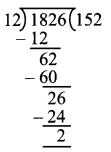Q = 152
R = 2
Divisor = 12
Dividend = 1,826

Dividend = Divisor × Q + R
L.H.S = 1,826
R.H.S = 12 × 152 + 2
= 1,824 + 2
= 1,824 + 2
= 1,826
∴ L.H.S = R.H.S
Hence, proved.

(b) 4,210 by 15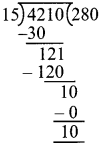Q = 280
R = 10
Divisor = 15
Dividend = 4,210

Dividend = Divisor × Q + R
L.H.S = 4,210
R.H.S = 280 × 15 + 10
= 4200 + 10
= 4210
∴ L.H.S = R.H.S

(c) 7,615 by 27Q = 280
R = 10
Divisor = 15
Dividend = 4,210

Dividend = Divisor × Q + R
L.H.S = 4,210
R.H.S = 280 × 15 + 10
= 4200 + 10
= 4210
∴ L.H.S = R.H.S(d) 9,885 by 46Q = 214
R = 41
Divisor = 46
Dividend = 9,885
Dividend = Divisor × Q × R
L.H.S = 9885
R.H.S = 46 × 214 + 41
= 9844 + 41
= 9885
L.H.S = R.H.S
Hence, checked

(e) 6,016 by 66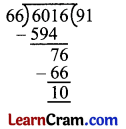Q = 91
R = 10
Divisor = 66
Dividend = 6,016
L.H.S = 6016
R.H.S = 91 × 66 + 10
= 6006 + 10
= 6016
L.H.S = R.H.S
Hence, checked

(f) 8,423 by 54